# Enhancing the efficiency of a DC-DC converter used for hybrid electrical vehicles to suit uphill and downhill terrains

Enhancing the efficiency of a DC-DC converter used for hybrid electrical vehicles to suit uphill and downhill terrains

Geetha Reddy EvuriSrinivasa Rao Gorantla T.Rama Subba Reddy

Electrical and Electronics Engineering, Vignan’s Foundation for Science, Technology and Research, Vadlamudi, Guntur, Andhra Pradesh 522213, India

Electrical and Electronics Engineering, VITS, Hyderabad, Telangana 501505, India

Corresponding Author Email:
gre.413@gmail.com
Page:
75-89
|
DOI:
https://doi.org/10.3166/EJEE.19.75-89
|
Accepted:
|
Published:
30 April 2017
| Citation

OPEN ACCESS

Abstract:

This paper presents a method to improve the efficiency of a DC-DC converter that is suitable for the hybrid electrical vehicles (HEV). In the recent past lot of work has been carried out in this area but a detailed study on various parameters was not carried out due to which most of the problems related with different environmental conditions were overseen. This study with detailed information on the load analysis over uphill and downhill terrains helps to understand the electrical vehicles performance for different load conditions along with different forces contributed due to the position of vehicle. These results are helpful to define the desired amounts of power requirements for the DC-DC converters to design an efficient HEV. In this work, all three types of converters using PI, PID and Fuzzy logic controller (FLC) were tested with a successful analysis of different parameters such as rise time, settling time, peak time, peak overshoot, steady state error and efficiency are listed in a tabular columns. The results show that the efficiency has been improved by using a FLC as compared to a PI and PID controllers to 93 %.

Keywords:

DC-DC converter, hybrid electric vehicle, Terrains, PI and PID

1. Introduction

In the recent past lot of work has been carried out in the area of hybrid vehicles, but a detailed study on various parameters was not carried out due to which most of the problems related with different environmental conditions were overseen. This paper with detailed information on the load analysis over uphill and downhill terrains which helps to understand the electrical vehicles performance for different load conditions along with different forces contributed due to the position of vehicle. A method to improve the efficiency of a DC-DC converter that is suitable for the hybrid electrical vehicles (HEV) has been proposed. A bidirectional DC-DC converter with closed loop controller with different modes of operations are being tested and simulated successfully after designing them practically. These results are helpful to define the desired amounts of power requirements for the DC-DC converters to design an efficient HEV. This paper also involves designing of different types of DC-DC converters like buck, boost, buck boost which can be used based on the type of load and required level of voltage. A PI, PID, Fuzzy controllers have been designed and different rules have been provided for fuzzy controller which works with different membership functions and a defuzzification method called centroid has been used. In this work, all three types of converters using PI, PID and Fuzzy logic controller (FLC) were tested with a successful analysis of different parameters such as rise time, settling time, peak time, peak overshoot, steady state error and efficiency.

2. Hybrid electric vehicles (HEV)

The usage of the electric vehicles (EV) and hybrid electric vehicles (HEV) are mostly discussed and popular topics, which are gaining attention of developed and developing nations to solve the problems related with environment and shortage of natural resources such as petroleum, coal and gas. The conventional vehicles movement is dependent on gasoline engine which generates the required force. A lot of maintenance is needed from filling the oil to changing the oil and regular repairs are quite essential. However, in an electric vehicle, electric motor provides the essential force to move the vehicle. But these vehicles need low maintenance, cheaper and are more energy-efficient. The efficiency of electric vehicles needs to be consistent when they are travelling in different terrain conditions. It is seen that the efficiency is always a challenging task for the designers of HEVs. In most of the modern HEVs, regenerative brakes (converts vehicle kinetic energy to electric energy) (Gao and Ehsani, 2001) are used to improve the efficiency. Here the electric energy will be stored into a super-capacitor or ultra-capacitor (UC) or a battery. In some cases internal combustion engines (Frank, 2000) are used to generate the electricity by spinning the generators or by directly powering the motors. The UC must be capable of storing high power densities and such property may create some kind of interference problems once the batteries are fully charged. To avoid such issues buck-boost converters were used as a replacement to UC for the recovery of energy while braking (Itani et al., 2016). The process of vehicle electrification started in 1950 by using hybrid energy storage systems (HESS. HESS is a composition of Li-Ion battery which in general used as an electrical source and in ultra-capacitors for braking systems and acceleration (Cegnar et al., 2004). Various authors worked on HESS to investigate its performance by using different types of controllers such as PI, PID and Fuzzy logic based. In this work, all such controllers are tested to propose an improved version of the HEV with enhanced efficiency to work with different terrains.

2.1. Recent work on DC-DC converters for HEVs

The demand for electric vehicles by both public and private transportation sectors made remarkable innovative milestones in the area of delivering energies at high power rates at the time of accelerating the engines and also to accept the high-power regeneration at the time of braking. Buck, boost and Buck-boost controllers are most popularly known in the market due to the ability to replace the ultra-capacitors. An auxiliary energy system (AES) proposed by Ortúzar used the ultra-capacitors and buck-boost converter to evaluate the EVs (Ortúzar, 2005). This arrangement could increase the power output from 40 kW to 85 kW. To accomplish this, experiment the author two algorithms were used based on heuristics and optimal control techniques, which are then applied to a neural network-based programming. The super-capacitor bank with a storage capacity of 100 Wh proposed by Lium was tested later using a bidirectional DC-DC converter with a bridge configuration and three inductors (Lium, 2007). This converter was based on standard intelligent power module, which is capable of switching 150 A at 600 V. This experiment was based on the boost system to drive different loads at high acceleration speeds. A constructive work was carried out by Pepper to study the advantages of discontinuous conduction mode (DCM) of operation against the continuous conduction mode (CCM) of operation in DC-DC converters revealed that the DCM operations do not hold the capacity of power flow in both directions. The DC-DC converter proposed in this work operated an EV with a high regulated voltage of 700 V as well.

Later, Martínez et al. proposed a buck-boost converter which can provide even fractional control for the EVs using non-integer order control to improve the performance of converter (Martínez et al., 2010). The authors in this work used ultra-capacitor-based feedback technique to overcome classical problems, such as energy losses due to battery usage and to obtain more accuracy, autonomy and efficiency. In an effort to design a fuel cell shaft power pack (FCSPP) for the small trucks Schaltz used the buck-boost converter to switch the voltages as per the requirement of the EV depending on the actual fuel cell power levels (Schaltz, 2010). This arrangement allowed a smooth transition between both the modes of operation. Later a plug-in HEV proposed by Hirota et al. used a new AC/DC converter which is suitable for supplying the power from a generalized commercial power system which we get in real life to the one suitable for onboard high-voltage battery also (Hirota et al., 2011). These converters demonstrated an efficiency of 90 % as compared with the conventional converters. However, the DC-DC converters are best suitable for avoiding the complexities of switching converters due to complexity, isolation, electronic noise, and high cost (Sakka et al., 2011). A DC-DC converter was proposed by Christen et al. for ultra-fast charging of an EV reported to achieve an efficiency of 99.5% for a wide operating range (Christen et al., 2012). Muller and Heinrich suggested adjusting the overall DC-link voltage for improving the efficiency of DC-DC converter at a level which is equal to optical operating point (Mueller and Heinrich, 2013). Apart from that at high operating points it helps to reduce the power losses for power electronics and electrical machines. Different types of buck, boost and buck-boost converters are provided with over current protection and frequency synchronization by Texas Instruments (Instruments, 2015). Variety of areas that benefit from DC-DC converters are given by a figure by Muller and Heinrich as shown in Fig. 1.

## 1.png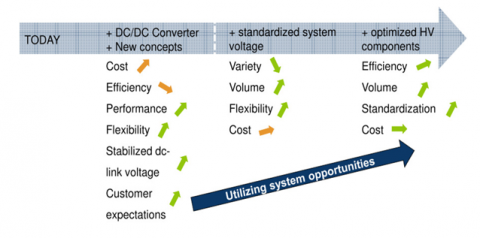Figure 1. The expected future path for DC-DC converter

In an attempt to provide minimum phase behavior and excellent dynamic performance with heavy loads Kumar et al. proposed a modified non-isolated bidirectional DC-DC converter for HEVs (Kumar et al., 2016). The application of the DC-DC converter was used between solar panels and battery by Elangovan et al. for lifting the DC voltages levels from the solar panels (Luo et al., 2010). The authors proposed an advanced DC-DC converter, naming it as super-lift converter (SLC), which is based on EVs.

3. Load analysis for uphill and downhill terrains

Consider a vehicle moving on a terrain with slop will be having the influence of various forces on it as shown in Figure 2. These forces are due to gravity, wind flow, inertia and rolling resistance need to be overcome by a motor of the truck. The forces acting on the driving wheels (Schaltz, 2010) is given by

$2 f _ { t } = \left( M _ { T r u c k } - 2 M _ { g w } \right) \dot { v } _ { \tau r u c k } + M _ { T r u c k } \cdot g \cdot \sin ( \alpha ) +$

$\sin \left( v _ { \tau r u c k } \right) M _ { T r u c k } \cdot g \cdot \cos ( \alpha ) \cdot C _ { r r } +$

$\sin \left( v _ { \tau r u c k } + v _ { w i n d } \right) \frac { 1 } { 2 } \rho _ { a i r } \cdot C _ { d r a g } \cdot A _ { f r o n t } \cdot g \left( v _ { \tau r u c k } + v _ { w i n d } \right) ^ { 2 }$       (1)

Here,

ft is traction force on driving wheels and is given by $\left( M _ { t r u c k } - 2 m _ { g w } \right) \dot { v } _ { \text {Truck} }$

fg is gravitational force on vehicle and is given by  $M _ { t r u c k } \cdot g$

fn is normal force of vehicle and is given by  $M _ { t r u c k } \cdot g \cdot \cos ( \alpha )$

frr is rolling resistance force of wheels and is given by $M _ { t r u c k } \cdot g \cdot \cos ( \alpha ) \cdot C _ { r r }$

fwind is force due to wind resistance and is given by $\frac { 1 } { 2 } \rho _ { a i r } \cdot C _ { d r a g } \cdot A _ { f r o n t } \cdot g \left( v _ { T r u c k } + v _ { w i n d } \right) ^ { 2 }$

$\alpha$  is the angle of driving surface

fl is inertial force on the vehicle

MTruck is total mass of the vehicle

## 2.png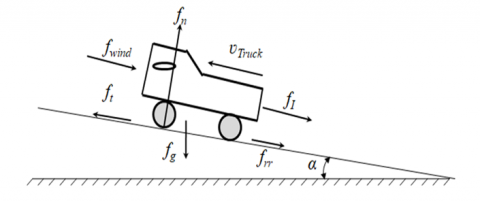Figure 2. Illustration of different forces acting on the truck

The relationships between different parameters such as shaft torque ($\tau _ { s }$ ), angular velocity ( $\omega _ { \mathrm { s } }$), traction force ( ft) and truck speed ( vTruck) are given by

$\tau _ { s } = J _ { g w } ^ { s } \frac { d \omega _ { s } } { d t } + B _ { v g w } ^ { s } \omega _ { s } + \operatorname { sign } \left( \omega _ { s } \right) \tau _ { c , g w } ^ { s } + \tau _ { t } ^ { s }$     (2)

$\tau _ { t } = G \tau _ { t } ^ { s }$     (3)

$f _ { t } = \frac { \tau _ { t } } { r _ { \omega } }$      (4)

$\omega _ { \omega } = \frac { \omega _ { s } } { G }$     (5)

$\mathbf { v } _ { \text {Truck} } = r _ { \omega } \omega _ { \omega }$     (6)

Here the parameters are given from the view of shaft side

$J _ { g w } ^ { S }$ is the equivalent moment of inertia of gear-box and wheel

$B _ { v , g w } ^ { s } \omega _ { s }$ is the equivalent viscous friction coefficient of the gear-box and wheel

$\tau _ { v , g w } ^ { s }$ is the equivalent coulomb torque of gear-box and wheel

G is the gear ratio

$\omega _ { \omega }$ is the wheel angular velocity

$\omega _ { s}$ is the shaft angular velocity

$\tau _ { t } ^ { s }$ is the traction force

In this experiment, a truck was considered to be travelling on a road with the slope, and calculated the shaft power when the maximum speed of the truck, and the load on the truck was tested for 0 and 1000 kg, the continuous consumption at 0% gradient was found to be 65% higher with a complete full load as compared with no loads. In case of a single power source, power source needs to provide two times the maximum power for the shafts and is calculated to be 16.65 kW. The results are shown in Fig. 3 by assuming the mass a driver to be 90 kg. The simulation results for slop of the road, truck speed, shaft torque and shaft power are shown in Fig. 3 for different masses of the loads.

## 3.png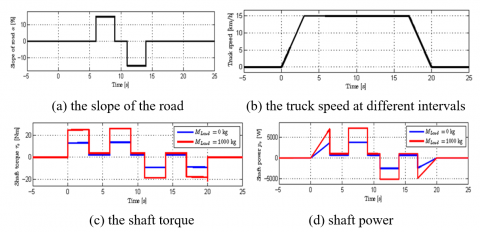Figure 3. The Simulation results for the truck with different loads of 0 and 1000 kg. Shows

From this experiment, it is observed that during the acceleration the battery current is very high and due to which there is a drop in inner resistance and voltages across the battery. However, the same battery voltage observed to increase when braking energy is given back as input to the battery.

4. Assessment of controllers for different terrains

In this work a test has been carried out for different types of terrains for a PI and Fuzzy Controllers to understand the EV behavior with different Controllers.

4.1. PI and PID control

A professional-integral (PI) controller is considered to be one of the special cases of professional-integral derivative (PID) controllers (Yu, 2006). Most of the control systems in the industry use these PID controllers to calculate the error values as a difference between measured process variable values and the desired set point values. For most of the closed loop operations in industrial automation the PID Controllers are widely used. A PID controller consists of a logic, function block, selector and sequence (Kecskés and Odry, 2014). The general approach to PID tuning is given below:

• At the initial stage set the integral gain to zero
• Now, increase the KP until a satisfactory response is observed; and
• Finally add the integral gain and then adjust KI so that the steady state error is eliminated

As compared to a PI controller, the fuzzy logic controller is completely based on a defined model of the system. But both will execute same control rules and standards (Kim and Lee, 2016). In this work, to understand the role of terrain resistance, road conditions and different terrains over an electric vehicle were examined and obtained the following results. To obtain the desired velocities by an HEV in different terrains most of the times the kinematic-based rough terrain control (KRTC) approach is used as suggested by Kim and Lee (Mendel et al., 2014). The simulation results for both uphill and downhill terrains are shown in Figure 3.

## 4.pngFigure 4. The simulation results for a PI controller (a) uphill terrain and (b) downhill terrains

In the uphill terrain response of a PI controller achieved a speed of 70 rad/s as shown in Figure 4 (a). But during the trip in rolling resistance drastic change is observed in uphill terrains. However, for downhill terrain the PI controller a speed of 58 rad/s is achieved as shown in Figure 4 (b). But in this process a sharp drop in speed is observed when rolling resistance is increasing.

4.2. Fuzzy logic control

A set of linguistic rules will define the basic controls of a fuzzy logic control (FLC) and the system will determine these rules (Ng et al., 2014). It does not require any kind of mathematical modeling since the rules are defined by the system itself. In general, a FLC consists of three major blocks: i) fuzzification, ii) interference engine, and iii) defuzzification.

On the other hand, the fuzzy controls are characterized by following five steps:

1. For each input and output apply seven fuzzy sets
2. For simplicity use triangular membership functions
3. For fuzzification use the continuous universe of discourse
4. Implementation using Mamdani “s” min operator; and
5. Finally, defuzzification using “height” method

FLC’s are well known to deliver appropriate results at the time of uncertainty and imprecision. Allows a layman to understand the functionality and develop own practical control algorithms with a human friendly thinking and perceptions. They outperformed traditional PID controllers and delivered similar results or even with more accuracy (Ng et al., 2014). A simple FLC block diagram is shown in Figure 5.

## 5.pngFigure 5. Shows the fuzzy logic controller

4.2.1. Fuzzification

Seven fuzzy subsets are used for fuzzification and for the linguistic variables different membership values will be assigned

1. Negative Big (NB)
2. Negative Medium (NM)
3. Negative Small (NS)
4. Zero (ZE)
5. Positive Small (PS)
6. Positive Medium (PM) and
7. Positive Big (PB).

In general, the partition of fuzzy subsets and shape of membership functions will adapt the shape up to appropriate desired system. Using the input scaling factors the value of input error, E(k) and change in error CE(k) are normalized. Different fuzzy rules used are given in Table 1.

Table 1. Fuzzy rules

 $\varepsilon \Delta \varepsilon$ NB NM NS ZE PS PM PB NB NB NB NB NB NM NS ZE NM NB NB NM NM NS ZE PS NS NB NM NS NS ZE PS PM ZE NB NM NS ZE PS PM PB PS NM NS ZE PS PS PM PB PM NS ZE PS PM PM PB PB PB ZE PS PM PB PB PB PB

In the deigned system, the input scaling factor is considered to be with the input values that are varying between -1 to +1. Also, it is considered that membership function will have a triangular shape and only one type of domain fuzzy subset will be available for any applied input. The simulation results of the membership functions are shown in Fig. 6.

## 6.png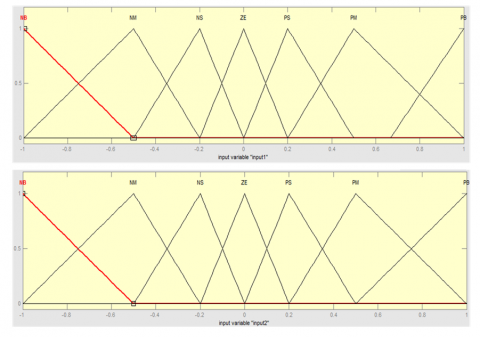Figure 6. Membership functions

4.2.2. Interference method

There are several compositions available such as Max–Min, Max-Dot, etc. but here Min method is applied. At this juncture the output membership function for any applied rule will be assigned by minimum and maximum operators.

4.2.3. Defuzzification

The role of defuzzification stage is needed due to the requirement of non-fuzzy value of controls. Height method is used to compute the FLC output and which later modifies the control output. The same FLC output will control inverter switches. To perform all these control parameters, all these parameters will be sensed and then compared with the defined references values. In this work, for fuzzification non-uniform fuzzifier was used. In case when the exacted values of error and change in error are very small, they are subjected to divide conversely. However, if they are very large, they are subjected to divide coarsely.

## 7.png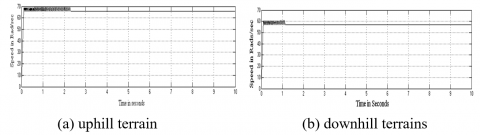Figure 7. The Simulation results for a fuzzy controller

In the uphill terrain response of a FLC achieved a speed of 70 rad/s (as shown in Figure 7 (a)). However, for downhill terrain FLC speed obtained was of 60 rad/s as shown in Figure 7 (b).

5. Measurement results for boost, buck and Buck-Boost converters

In this study, all the three types of converters are designed and tested for proposing an improved version of converter to improve the efficiency.

5.1. Boost converter

This is condered to be a step-up converter for DC-to-DC power conversion. In the designed boost converter, the efficiency for PI, PID and FLC are marginally improved as shown in Table 3. The corresponding values of rise time, settling time, peak time, peak overshoot, steady state error and efficiency are also shown in the table.

Table 2. Boost converter

 PI PID FUZZY Rise Time, tr (sec) 0.09 0.06 0.001 Settling Time, ts (sec) 0.3 0.22 0.08 Peak Time, tp (sec) 0.17 0.11 0.002 Peak Overshoot (%) 7.2 8.3 13.3 Steady State Error, (ESS) 0.03 0.02 0.02 Efficiency (%) 85 87.3 89 THD (%) 5.57 3.41 2.73

The obtained results are given below for different converters are as listed in Table 2 with the plots as shown in Figure 8.

## 8.pngFigure 8. Boost with

The applied input votage at boost converter input is boosted to 50 V at the output from the designed circuit. For each converter all the controllers (i.e. PI, PID, FLC) are applied and the results are quantified. The efficiency seems to be increasing using the FLC to 85 to 89 % as compared with PI and PID controllers.

5.2. Buck converter

This is a step-down converter for DC-to-DC power conversion. In the designed buck conversion, the efficiency for PI, PID and FLC are improved as shown in Table 3. The corresponding values of rise time, settling time, peak time, peak overshoot, steady state error and efficiency are also shown in the table.

Table 3. Buck converter

 PI PID FUZZY Rise Time, tr (sec) 0.02 0.01 0.012 Settling Time, ts (sec) 0.22 0.13 0.04 Peak Time, tp (sec) 0.03 0.02 0.04 Peak Overshoot (%) 10.4 11.3 0 Steady State Error, (ESS) 0.03 0.02 0.01 Efficiency (%) 87 88.5 89.3 THD (%) 5.99 4.65 3.21

The obtained results are given below for different converters are as listed in Table 3 with the plots as shown in Figure 9.

## 9.png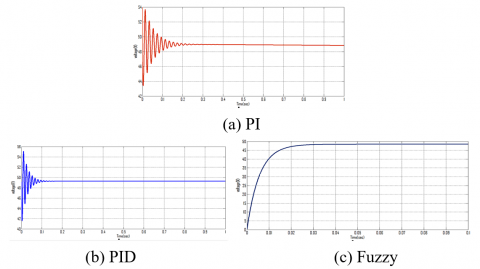Figure 9. Buck with

The buck converter designed in this work reduced the applied input votage 50 V to a low value at the output from. The efficiency seems to be increasing using the FLC to 85 to 89 % as compared with PI and PID controllers.

5.3. Buck-Boost converter

This is DC-to-DC power converter which provides an output voltage with required levels of voltage magnitudes based on the circuit requirements. In the designed buck-boost converter the efficiency for PI, PID and FLC increased from 90 to 93 % as shown in Table 4. The corresponding values of rise time, settling time, peak time, peak overshoot, steady state error and efficiency are also shown in the table.

Table 4. Buck-Boost converter

 PI PID FUZZY Rise Time, tr (sec) 0.01 0.02 0.06 Settling Time, ts (sec) 0.17 0.1 0.02 Peak Time, tp (sec) 0.03 0.03 0.02 Peak Overshoot (%) 10 7 0 Steady State Error, (ESS) 0.7 0.6 0.43 Efficiency (%) 90.1 90.5 93 THD (%) 4.11 3.24 2.16

The obtained results are given below for different converters are as listed in Table 4 with the plots as shown in Figure 10.

## 10.pngFigure 10. Buck-Boost with

5.4. Discussions

Boost, Buck and Buck-boost converter operating with PI, PID, and FUZZY controllers proposed in this work are designed practically and successfully simulated using MATLAB tools. Though the boost converter with fuzzy controller has a good rise time of 0.001 sec, the percentage of total harmonic distortion (THD) is 2.73 and efficiency is 89%. In case of buck converter with fuzzy controller the efficiency has been improved to 89.3%.  Fuzzy controller base buck-boost converter has given a very good performance as compared with PI and PID controllers. Though it has slightly longer rise time as compared with PI and PID controllers. In this, the THD has been reduced to 2.16% and with an improved efficiency of 93%.

6. Conclusions

In this work, a bidirectional DC-DC converter with closed loop controller with different modes of operations are being tested and simulated successfully after designing them practically. In the case of closed loop systems-based controllers the results observed for fuzzy controllers are providing better results when compared with both PI and PID controllers. Zero peak overshoot and lesser steady state error were obtained in this research as a main contribution to the existing controller systems. As a result, the overall THD found to be reduced which in turn supported to enhance the overall efficiency of the system up to 93%.

The converters based on Neuro fuzzy controller are under test as a part of future work. In this work a comparison between fuzzy and neuro fuzzy controllers will be made to establish a clear understanding of the proposed work.

References

Cegnar E. J., Hess H. L., Johnson B. K. (2004). A purely ultra capacitor energy storage system hybrid electric vehicles utilizing a based DC-DC boost converter. In Applied Power Electronics Conference and Exposition, APEC'04. Nineteenth Annual IEEE, Vol. 2, pp. 1160-1164. https://doi.org/10.1109/APEC.2004.1295969

Christen D., Tschannen S., Biela J. (2012). Highly efficient and compact DC-DC converter for ultra-fast charging of electric vehicles. In Power Electronics and Motion Control Conference (EPE/PEMC), 2012 15th International. https://doi.org/10.1109/EPEPEMC.2012.6397481

Frank A. A. (2000). Control method and apparatus for internal combustion engine electric hybrid vehicles. U.S. Patent 6,054,844, Vol. 25.

Gao Y., Ehsani M. (2001). Electronic braking system of EV and HEV---integration of regenerative braking. Automatic Braking Force Control and ABS, No. 2001-01-2478.

González R. M., Monte Y. B., Saldes A. G., García H. M. (2010). Buck-boost converter with fractional control for electric vehicles.

Hirota M., TakeshiBaba X. Z., Kazuhiko N. I. I., Ohashi S., Ariyoshi T., Fujikawa H. (2011). Development of New AC/DC converter for PHEVs and EVs. Sei Technical Review.

Instruments T. (2015). Hybrid and electric vehicle solutions guide.

Itani K., Bernardinis D. A., Khatir Z., Jammal A., Oueidat M. (2016). Regenerative braking modeling, control, and simulation of a hybrid energy storage system for an electric vehicle in extreme conditions. IEEE Transactions on Transportation Electrification, Vol. 2, No. 4, pp. 465-479. https://doi.org/10.1109/TTE.2016.2608763

Kecskés I., Odry P. (2014). Optimization of PI and Fuzzy-PI controllers on simulation model of Szabad (ka)-II walking robot. International Journal of Advanced Robotic Systems, Vol. 11, No. 11, pp. 186.

Kim J., Lee J. (2016). A kinematic-based rough terrain control for traction and energy saving of an exploration rover. In Intelligent Robots and Systems (IROS), IEEE/RSJ International Conference on, pp. 3595-3600. https://doi.org/10.1109/IROS.2016.7759529

Kumar B. V., Singh R. K., Mahanty R. (2016). A modified non-isolated bidirectional DC-DC converter for EV/HEV's traction drive systems. In Power Electronics, Drives and Energy Systems (PEDES), IEEE International Conference on, pp. 1-6. https://doi.org/10.1109/PEDES.2016.7914345

Lium F. (2007). 30 kW Power Boost System for Drive Trains for Electric Vehicles Based on Super capacitor Technologies.

Luo Y., Chen Y. Q., Wang C. Y., Pi Y. G. (2010). Tuning fractional order proportional integral controllers for fractional order systems. Journal of Process Control, Vol. 20, No.7, pp. 823-831. https://doi.org/10.1016/j.jprocont.2010.04.011

Mendel J., Hagras H., Tan W. W., Melek W. W., Ying H. (2014). Introduction to type-2 fuzzy logic control: theory and applications. John Wiley & Sons. https://doi.org/10.1007/978-3-642-24663-0_1

Mueller K., Heinrich R. (2013). Modern high voltage drive train architecture to accommodate the needs for a variety of components for future automotive applications. In CoFAT.

Ng T. S., Hung Y. S. (2014). Special issue on safety, reliability and applications of emerging intelligent control technologies. Elsevier.

Ortúzar M. E. (2005). Design, implementation and evaluation of an auxiliary energy system for electric vehicles, based on ultra capacitors and buck-boost converter. Santiago de Chile: Pontificia Universidad Catolica de Chile.

Pepper M. (2008). Bi-directional Dcm Dc-to-dc Converter For Hybrid Electric Vehicles. PESC Record-IEEE Annual Power Electronics Specialists Conference. https://doi.org/10.1109/PESC.2008.4592425

Sakka A. M., Van Mierlo J., Gualous H. (2011). DC/DC converters for electric vehicles. In Electric Vehicles-Modelling and Simulations.

Schaltz E. (2010). Design of a fuel cell hybrid electric vehicle drive system. Department of Energy Technology, Aalborg University.

Yu C. C. (2006). Auto tuning of PID controllers: A relay feedback approach. Springer Science & Business Media.